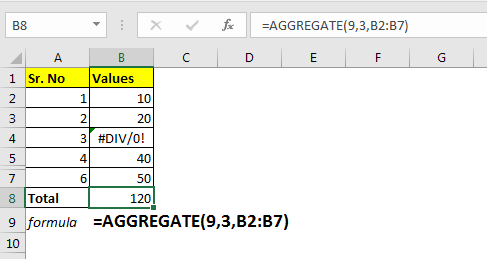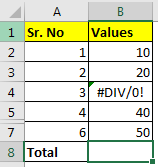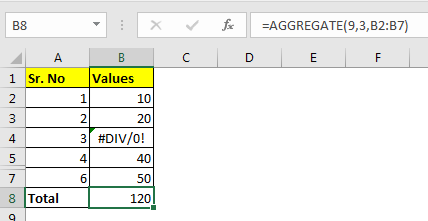# How to Use Excel AGGREGATE Function

The Excel AGGREGATE function of excel is an advanced version of Excel SUBTOTAL function. The AGGREGATE function was introduced in Excel 2010. It used to do simple operations on data set, like SUM, AVERAGE, MAX, etc same as SUBTOTAL.Then why use AGGREGATE function? The reason is, while the SUBTOTAL function consists of only 11 operations AGGREGATE handles 19 operations with more control. With control, I mean that you can control which values to be calculated in range or database. We will see how soon in this article.
Syntax of AGGREGATE Function

=AGGREGATE(function num, options, array,[k])    (Array Form)
=AGGREGATE(function num, options, ref1, ref2...) (Reference Form)

There are two forms of AGGREGATE Function in excel. Array Form and Reference Form. When we want to supply data as a range (eg A1:A3), we use array form. When we need to supply different references (eg, A1, B3, C11 etc) than we use Reference Form.
Function Num: From number 1 to 19, each number is associated with some operation. We provide the number of the function we want to use on range or database. Here is the list.

 Function Num Function Name 1 AVERAGE 2 COUNT 3 COUNTA 4 MAX 5 MIN 6 PRODUCT 7 STDEV.S 8 STDEV.P 9 SUM 10 VAR.S 11 VAR.P 12 MEDIAN 13 MODE.SNGL 14 LARGE 15 SMALL 16 PERCENTILE.INC 17 QUARTILE.INC 18 PERCENTILE.EXC 19 QUARTILE.EXC

Options: The control I was talking about, this options is that control. It enables you to choose, how you want to calculate. What you want to consider while calculating and what not. The list of available options is as below.

 Num Options 0 Ignore nested SUBTOTAL and AGGREGATE functions 1 Ignore nested SUBTOTAL, AGGREGATE functions, and hidden rows 2 Ignore nested SUBTOTAL, AGGREGATE functions, and error values 3 Ignore nested SUBTOTAL, AGGREGATE functions, hidden rows & error values 4 Ignore nothing 5 Ignore hidden rows 6 Ignore error values 7 Ignore hidden rows and error values

Array or Ref: This the range on which you want to perform operations. It can be a database, single-cell or series of unlinked cells.

[k]: It is an optional argument. It is must be used with the functions that require a key. Like SMALL, LARGE,  etc.
Let’s see an example to make things clear.

### Example: Sum visible range, ignore errors using AGGREGATE function

Here I have a small set of numbers that I want to sum. Now the condition is, I want to sum values that are visible only. I want to ignore any errors, SUBTOTAL and AGGREGATE formulas in-between range.Write this formula in cell B8.

=AGGREGATE(9,3,B2:B7)

Above AGGREGATE formula will return the correct answer as we expect.If you use the SUBTOTAL function, it will handle the hidden rows but it will fail to handle errors.

You can also get nth SMALLEST or nth LARGEST value too using AGGREGATE formula in excel.

=AGGREGATE(14,3,B2:B7,2)

The above formula will return the second largest value in range B2:B7. Which is 40 here.

=AGGREGATE(4,3,B7,B3)

The above AGGREGATE formula is reference type and used to get max value between B7 and B3.

Notes:

• If a function does not require a key [k], and, AGGREGATE will return a #VALUE error.
• If a function requires a key [k], and you don’t provided it, AGGREGATE will return a #VALUE error.

Related Articles:

How to Use SUBTOTAL Function in Excel

How to use the Excel LOG10 function

How to use the IMEXP Function in Excel

How to use the IMCONJUGATE Function in Excel

How to use the IMARGUMENT Function in Excel

Popular Articles

Edit a dropdown list

If with conditional formatting

If with wildcards

Vlookup by date

Terms and Conditions of use

The applications/code on this site are distributed as is and without warranties or liability. In no event shall the owner of the copyrights, or the authors of the applications/code be liable for any loss of profit, any problems or any damage resulting from the use or evaluation of the applications/code.